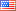# Revision history [back]

### Is there an example of how i could write a polynomial as a product of linear factors

Is there an example of how i could write a polynomial as a product of linear factors.2 Update tagsMike Hansen4068 ●24 ●48 ●89

### Is there an example of how i could write a polynomial as a product of linear factors

Is there an example of how i could write a polynomial as a product of linear factors.3 retagged

### Is there an example of how i could write a polynomial as a product of linear factors

Is there an example of how i could write a polynomial as a product of linear factors.